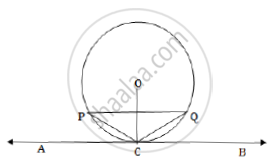# Prove that the tangent drawn at the mid-point of an arc of a circle is parallel to the chord joining the end points of the arc. - Mathematics

Prove that the tangent drawn at the mid-point of an arc of a circle is parallel to the chord joining the end points of the arc.

#### Solution

In the figure, C is the midpoint of the minor arc PQ, O is the centre of the circle and AB is tangent to the circle through point C. We have to show that the tangent drawn at the midpoint of the arc PQ of a circle is parallel to the chord joining the end points of the arc PQ.

We will show that PQ || AB.

It is given that C is the midpoint point of the arc PQ.

So, arc PC = arc CQ.

PC = CQThis shows that ΔPQC is an isosceles triangle.

Thus, the perpendicular bisector of the side PQ of ΔPQC passes through vertex C. The perpendicular bisector of a chord passes through the centre of the circle. So the perpendicular bisector of PQ passes through the centre O of the circle. Thus perpendicular bisector of PQ passes through the points O and C.

∴PQ ⊥ OC

AB is the tangent to the circle through the point C on the circle.

AB ⊥ OC

The chord PQ and the tangent PQ of the circle are perpendicular to the same line OC.

∴PQ || AB.

Concept: Concept of Circle - Centre, Radius, Diameter, Arc, Sector, Chord, Segment, Semicircle, Circumference, Interior and Exterior, Concentric Circles
Is there an error in this question or solution?